Open in App
Not now

# Combine two images in MATLAB

• Difficulty Level : Expert
• Last Updated : 22 Sep, 2021

In this article, we will learn how to combine two images of the same size in MATLAB.

Now, let’s suppose we have been given two colored images of different sizes. One image has the main figure on the left side and the other has one on the right side. We need to combine the two images such that both figures appear on them.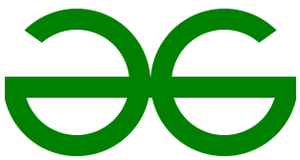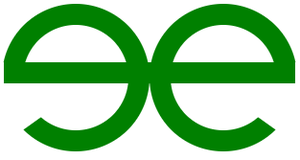We need to combine them.

Step 1: Make left half of right_side_image 0 intensity image(black). Also, make right half of left_side_image 0 intensity region.

Syntax:

image_var = imread(” image path here “);

var_name = rgb2gray (image_var);

size( image_var )

imshow( var_name , [ ]);

or

imtool( var_name, [ ]);

Access half columns :

image_var( :, 1:150);

Example I:

## Matlab

 `% MATLAB code for read the left ``% and right side of images.``left=imread(``"logo_left.png"``);``right=imread(``"logo_right.png"``);`` ` `% Convert them into grayscale images.``left=rgb2gray(left);``right=rgb2gray(right);`` ` `% Display the gray scaled images.``imtool(left, []);``imtool(right, []);`` ` `% Display the size of both images, then choose resize.``size(left) ``% size of left is= 160   300     3``size(right) ``% size of right is= 160   300     3`` ` `% Make other half as 0 intensity region.``left(:, 150:300)=0;``right(:, 1:150)=0;`` ` `% Display both images.``imtool(left, []);``imtool(right, []);`

Output: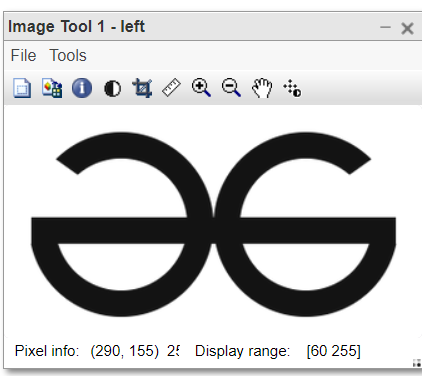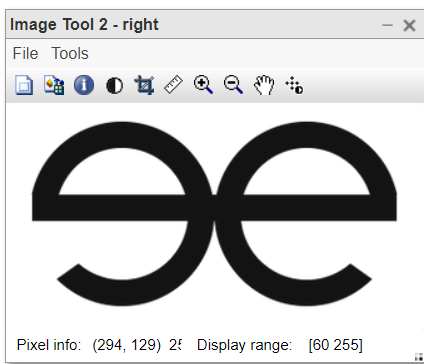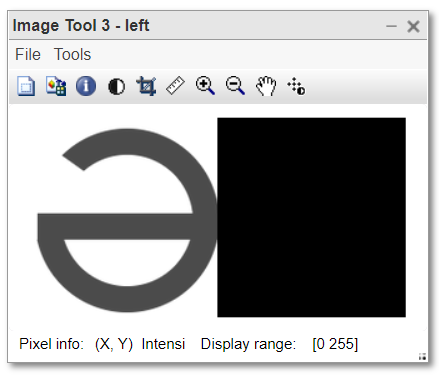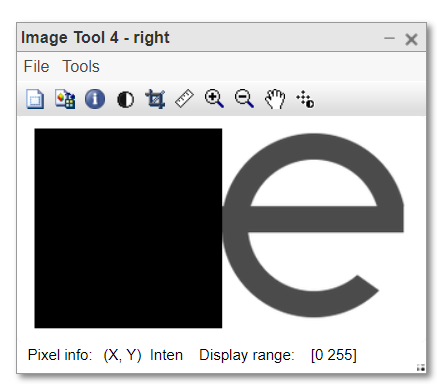• In the above program first, we read the left and right images.
• Converted the colored images into grayscale images.
• Displayed the images.
• Displayed the sizes of both images.
• Converted other half intensity as 0. made half part dark.
• Displayed modified images.

Step 2: Combine the left and right parts.

Syntax:

new_image = image_1 + image_2

// condition: both images must be of same size and type.

Example II:

## Matlab

 `% MATLAB code for image combine ``% read the left and right sided images.``left=imread(``"logo_left.png"``);``right=imread(``"logo_right.png"``);`` ` `% Convert them into grayscale images.``left=rgb2gray(left);``right=rgb2gray(right);`` ` `% Display the gray scaled images.``imtool(left, []);``imtool(right, []);`` ` `% Display the size of both images, then choose resize.``size(left) ``% size of left is= 160   300     3``size(right) ``% size of right is= 160   300     3`` ` `% Make other half as 0 intensity region.``left(:, 150:300)=0;``right(:, 1:150)=0;`` ` `% Display both images.``imtool(left, []);``imtool(right, []);`` ` `% Combine left and right images and display.``combined=uint8(left+right);``imtool(combined,[]);`

Output: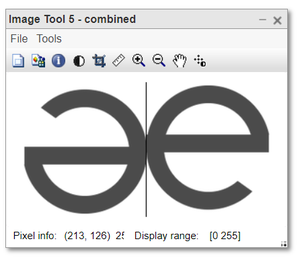The output image is the combination of two images.

My Personal Notes arrow_drop_up